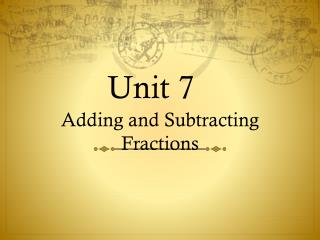DownloadDownload PresentationDownload Presentation- - - - - - - - - - - - - - - - - - - - - - - - - - - E N D - - - - - - - - - - - - - - - - - - - - - - - - - - -
##### Presentation Transcript

1. Question #1 • 1 + 2 • 5 3

2. Question #1 • 1 + 2 = 13 • 5 3 15

3. Question #2

5. Question #3

6. Answer #3 Answer is = 2 = 3 = 15 = 33

7. Question #4

9. Question #5

10. Answer #5 Answer is 1/3 + 1/6 2/6 + 1/6 = 3/6 or 1/2

11. Question #6

13. Question #7

14. Answer #7 Answer is 3/6 - 4/12 6/12 - 4/12 = 2/12 = 1/6

15. Question #8

17. Question #9 What is the result, in lowest terms, of subtracting the fractions represented by the shaded pieces of the figure?

18. Question #9 What is the result, in lowest terms, of subtracting the fractions represented by the shaded pieces of the figure? Answer is 3/6 – 1/6 = 2/6 = 1/3

19. Question #10

20. Answer #10 Answer is 1 1/6 + 1 = 2 1/6

21. Question #11

22. Answer #11 Answer is 1 4/5 + 2 1/5 = 3 5/5 = 4

23. Question #12 Solve

25. Question #13 Solve

27. Question #14

28. Question #14 Answer is 1 ¼ + 1 ¾ = 2 4/4 = 3

29. Question #15

30. Answer #15 Answer is 2 1/6 + 3 3/6 = 5 4/6 = 5 2/3

31. Question #16

32. Answer #16 Answer is 2 ¾ - 1 ¼ = 1 2/4 = 1 1/2

33. Question #17 Solve

34. Question #17 Answer is 2 2/5

35. Question #18 Solve